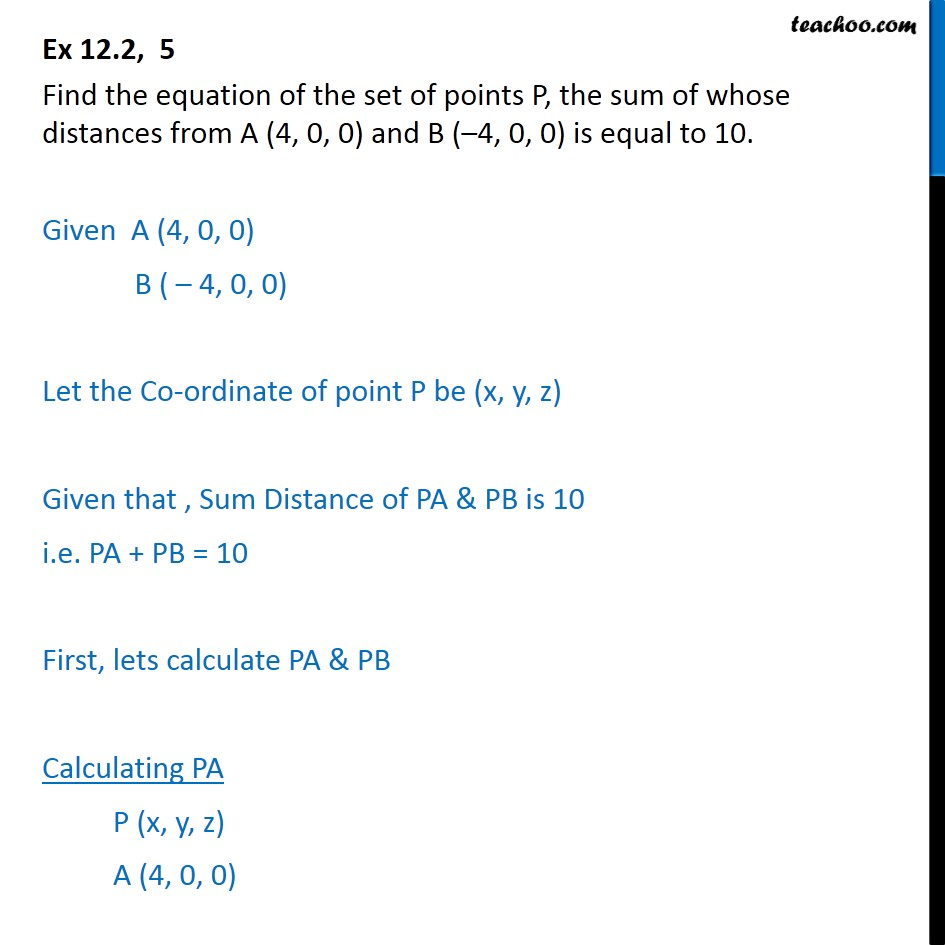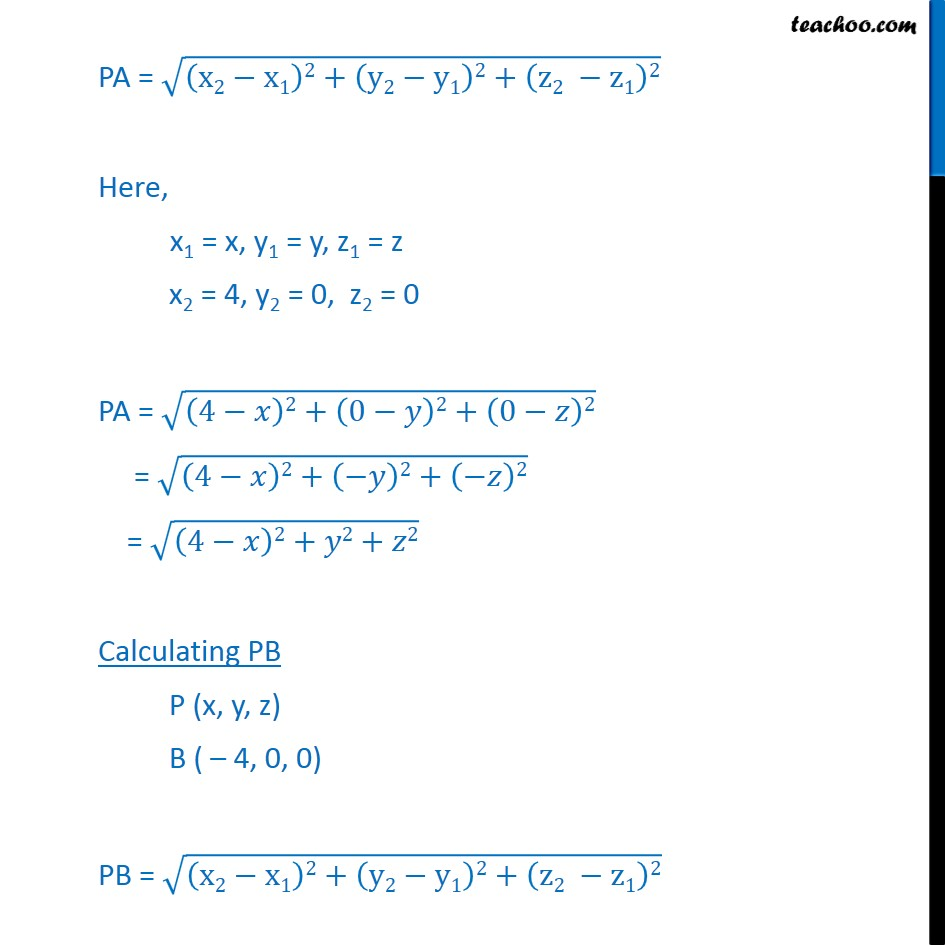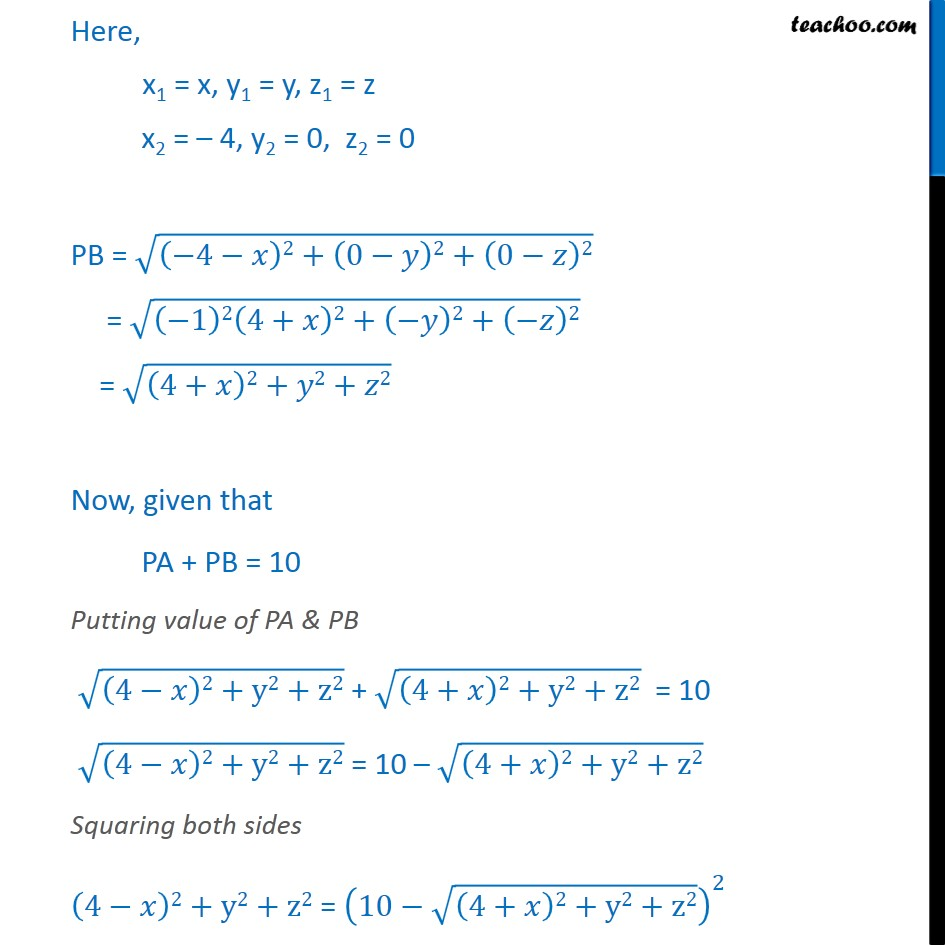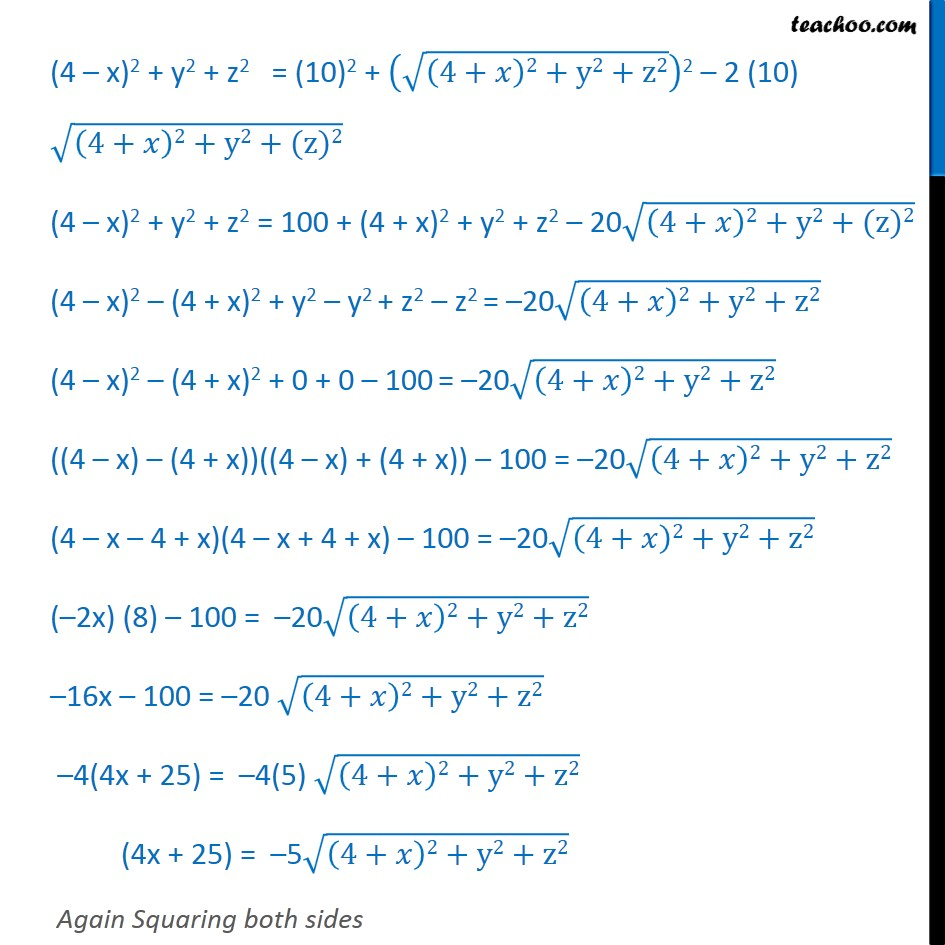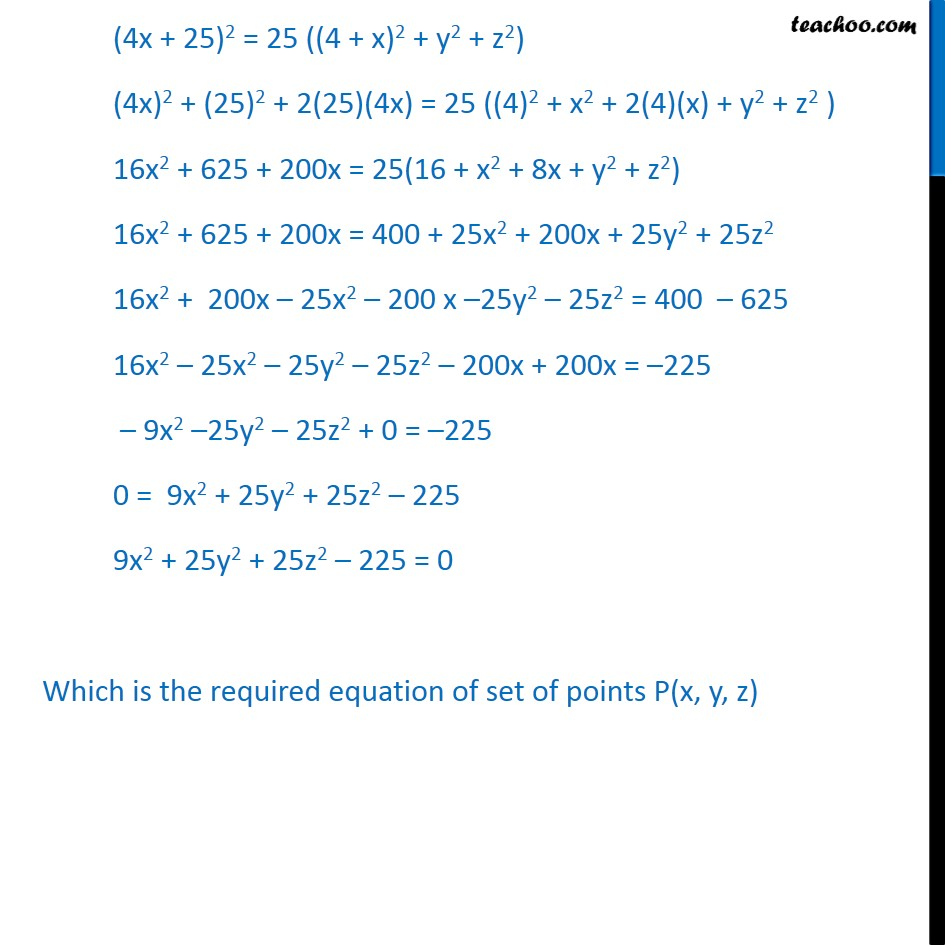1. Chapter 12 Class 11 Introduction to Three Dimensional Geometry
2. Serial order wise
3. Ex 12.2

Transcript

Ex 12.2, 5 Find the equation of the set of points P, the sum of whose distances from A (4, 0, 0) and B (–4, 0, 0) is equal to 10. Given A (4, 0, 0) B ( – 4, 0, 0) Let the Co-ordinate of point P be (x, y, z) Given that , Sum Distance of PA & PB is 10 i.e. PA + PB = 10 First, lets calculate PA & PB Calculating PA P (x, y, z) A (4, 0, 0) PA = √((x2−x1)2+(y2−y1)2+(z2 −z1)2) Here, x1 = x, y1 = y, z1 = z x2 = 4, y2 = 0, z2 = 0 PA = √((4−𝑥)2+(0−𝑦)2+(0−𝑧)2) = √((4−𝑥)2+(−𝑦)2+(−𝑧)2) = √((4−𝑥)2+𝑦2+𝑧2) Calculating PB P (x, y, z) B ( – 4, 0, 0) PB = √((x2−x1)2+(y2−y1)2+(z2 −z1)2) Here, x1 = x, y1 = y, z1 = z x2 = – 4, y2 = 0, z2 = 0 PB = √((−4−𝑥)2+(0−𝑦)2+(0−𝑧)2) = √((−1)2(4+𝑥)2+(−𝑦)2+(−𝑧)2) = √((4+𝑥)2+𝑦2+𝑧2) Now, given that PA + PB = 10 Putting value of PA & PB √((4−𝑥)2+y2+z2) + √((4+𝑥)2+y2+z2) = 10 √((4−𝑥)2+y2+z2) = 10 – √((4+𝑥)2+y2+z2) Squaring both sides (4−𝑥)2+y2+z2 = (10−√((4+𝑥)2+y2+z2))^2 (4 – x)2 + y2 + z2 = (10)2 + (√((4+𝑥)2+y2+z2))2 – 2 (10) √((4+𝑥)2+y2+(z)2) (4 – x)2 + y2 + z2 = 100 + (4 + x)2 + y2 + z2 – 20√((4+𝑥)2+y2+(z)2) (4 – x)2 – (4 + x)2 + y2 – y2 + z2 – z2 = –20√((4+𝑥)2+y2+z2) (4 – x)2 – (4 + x)2 + 0 + 0 – 100 = –20√((4+𝑥)2+y2+z2) ((4 – x) – (4 + x))((4 – x) + (4 + x)) – 100 = –20√((4+𝑥)2+y2+z2) (4 – x – 4 + x)(4 – x + 4 + x) – 100 = –20√((4+𝑥)2+y2+z2) (–2x) (8) – 100 = –20√((4+𝑥)2+y2+z2) –16x – 100 = –20 √((4+𝑥)2+y2+z2) –4(4x + 25) = –4(5) √((4+𝑥)2+y2+z2) (4x + 25) = –5√((4+𝑥)2+y2+z2) Again Squaring both sides (x + 25)2 = ( – 5)2 (√((4+𝑥)2+y2+z2))2 (4x + 25)2 = 25 ((4 + x)2 + y2 + z2) (4x)2 + (25)2 + 2(25)(4x) = 25 ((4)2 + x2 + 2(4)(x) + y2 + z2 ) 16x2 + 625 + 200x = 25(16 + x2 + 8x + y2 + z2) 16x2 + 625 + 200x = 400 + 25x2 + 200x + 25y2 + 25z2 16x2 + 200x – 25x2 – 200 x –25y2 – 25z2 = 400 – 625 16x2 – 25x2 – 25y2 – 25z2 – 200x + 200x = –225 – 9x2 –25y2 – 25z2 + 0 = –225 0 = 9x2 + 25y2 + 25z2 – 225 9x2 + 25y2 + 25z2 – 225 = 0 Which is the required equation of set of points P(x, y, z)

Ex 12.2